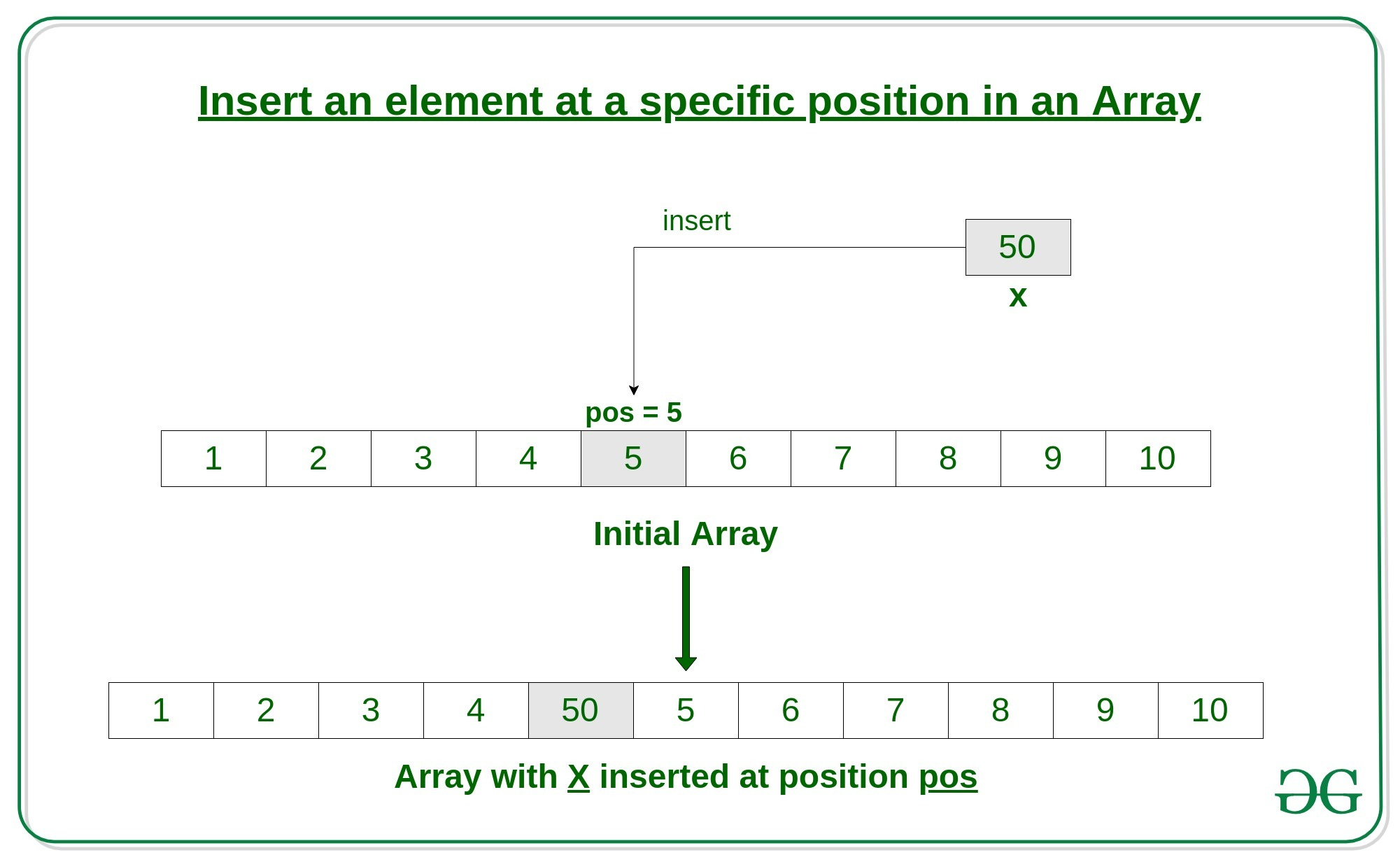Open in App
Not now

# C program to Insert an element in an Array

• Difficulty Level : Easy
• Last Updated : 12 Sep, 2022

Given an array arr of size n, this article tells how to insert an element x in this array arr at a specific position pos.

An array is a collection of items stored at contiguous memory locations. In this article, we will see how to insert an element in an array in C.Follow the below steps to solve the problem:

• First get the element to be inserted, say x
• Then get the position at which this element is to be inserted, say pos
• Then shift the array elements from this position to one position forward(towards right), and do this for all the other elements next to pos.
• Insert the element x now at the position pos, as this is now empty.

Below is the implementation of the above approach:

## C

 `// C Program to Insert an element` `// at a specific position in an Array`   `#include `   `int` `main()` `{` `    ``int` `arr = { 0 };` `    ``int` `i, x, pos, n = 10;`   `    ``// initial array of size 10` `    ``for` `(i = 0; i < 10; i++)` `        ``arr[i] = i + 1;`   `    ``// print the original array` `    ``for` `(i = 0; i < n; i++)` `        ``printf``(``"%d "``, arr[i]);` `    ``printf``(``"\n"``);`   `    ``// element to be inserted` `    ``x = 50;`   `    ``// position at which element` `    ``// is to be inserted` `    ``pos = 5;`   `    ``// increase the size by 1` `    ``n++;`   `    ``// shift elements forward` `    ``for` `(i = n - 1; i >= pos; i--)` `        ``arr[i] = arr[i - 1];`   `    ``// insert x at pos` `    ``arr[pos - 1] = x;`   `    ``// print the updated array` `    ``for` `(i = 0; i < n; i++)` `        ``printf``(``"%d "``, arr[i]);` `    ``printf``(``"\n"``);`   `    ``return` `0;` `}`

Output

```1 2 3 4 5 6 7 8 9 10
1 2 3 4 50 5 6 7 8 9 10
```

Time Complexity: O(N), Where N is the number of elements in the array
Auxiliary Space: O(1)

My Personal Notes arrow_drop_up
Related Articles